Reach Us+32-10-28-02-25
Thermal Diffusive Free Convective Radiating Flow Over an Impulsively Started Vertical Porous Plate in Conducting Field | OMICS International
Journal of Physical Mathematics
All submissions of the EM system will be redirected to Online Manuscript Submission System. Authors are requested to submit articles directly to Online Manuscript Submission System of respective journal.

# Thermal Diffusive Free Convective Radiating Flow Over an Impulsively Started Vertical Porous Plate in Conducting Field

Kumar VR1, Raju MC1*, Raju GSS2 and Varma SVK3

1Department of Mathematics, Annamacharya Institute of Technology and Sciences, India

2Department of Mathematics, JNTUA College of Engineering, India

3Department of Mathematics, S.V. University, India

*Corresponding Author:
Raju MC
Department of Mathematics, Annamacharya
Institute of Technology and Sciences, India
Tel: 009457759877
E-mail: [email protected]

Received Date: January 11, 2016; Accepted Date: January 31, 2016; Published Date: Feruary 04, 2016

Citation: Kumar VR, Raju MC, Raju GSS, Varma SVK (2016) Thermal Diffusive Free Convective Radiating Flow Over an Impulsively Started Vertical Porous Plate in Conducting Field. J Phys Math 7:156. doi:10.4172/2090-0902.1000156

Copyright: © 2016 Kumar VR, et al. This is an open-access article distributed under the terms of the Creative Commons Attribution License, which permits unrestricted use, distribution, and reproduction in any medium, provided the original author and source are credited.

Visit for more related articles at Journal of Physical Mathematics

#### Abstract

In this manuscript we have studied the laminar convective heat and mass transfer flow of an incompressible, viscous, electrically conducting fluid over a fluid over an impulsively started vertical plate with conduction-radiation embedded in a porous medium in the occurrence of transverse magnetic field. An exact solution is derived by solving the dimensionless main coupled partial differential equations using Laplace transform technique. The properties of important physical parameters on the velocity, temperature, concentration, Skin friction, Sherwood number and Nusselt number have been studied through graphs.

#### Keywords

MHD; Porous medium; Thermal diffusion; Thermal radiation; Shear stress; Nusselt number and Sherwood number

#### Nomenclature

C/ : Species concentration fluid

Cp : Specific heat at constant pressure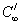: Concentration of the fluid for away from the plate: Concentration level near the plate/wall

D: Chemical molecular diffusivity

g: Acceleration due to gravity

Gr: Thermal Grashof number

Gm: modified Grashof number

Kr : Permeability parameter

M: Hartmann number

Nu: Nusselt number

Pr: Prandtl number

S0: Soret number

Sh: Sherwood number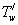: Fluid temperature at the surface

u: Dimensional velocity components

Sc: Schmidt number

T/: Temperature

u0: Plate velocity

β : coefficient of volume expansion for heat transfer

θ: Dimensional fluid

n: Kinematic viscosity

σ : Electrical conductivity

C: Dimensionless species concentration

βc: Coefficient of volume expansion for mass transfer

κ: Thermal conductivity

ρ : Density

τ: Shearing stress

w: Condition on the wall

∞ : Free stream condition

#### Mathematical Formulations

The laminar convective heat as well as mass transfer flow of an incompressible, viscous, electrically conducting fluid over an impulsively started vertical plate among conduction-radiations embedded in a porous medium in presence of transverse magnetic field has been studied. The x/ axis is taken the length of plate in the vertical upward direction and the y/ axis is taken normal to the plate. A transverse magnetic field of identical strength B0 is assumed to be applied normal to the plate. It is also implicit that the thermal radiation along the plate and viscous dissipation is implicit to be negligible. The induced magnetic field and viscous dissipation is understood to be negligible. Initially it is assumed that the plate and fluid are at same temperature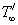in the stationary situation with concentration levelat all the points. At time,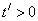the plate is specified an impulsive motion in its own plane with velocity u0. The temperature of the plate and the concentration stage are also raised toand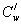. They are maintained at the similar level for all time. Then under the above assumption the unsteady flow with usual Boussinesq’s estimate is governed by the following equations.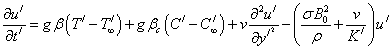(1)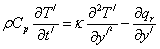(2)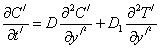(3)

The initial and boundary conditions are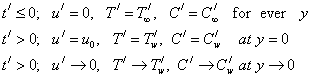(4)

The radiation heat flux term is simplified by making use of the Rosseland approximation  as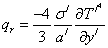(5)

Where σ/ and a/ are the Stefan-Boltzmann steady and the mean absorption coefficient respectively. It should be noted that by using the Roseland approximation, we limit our investigation to optically thick fluids. It temperature differences within the flow are sufficiently small, such that T/4 may be expressed as a linear function of the temperature, then the Taylors series for T/4 and, after neglecting higher order terms is given by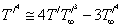(6)

Substitute (5) and (6) in (2) we have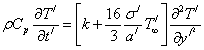(7)

Let us introduce the following non-dimensional terms in (1), (7) and (3)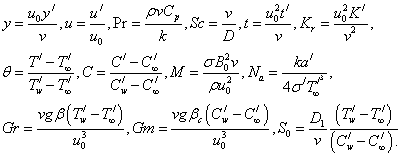(8)

Hence the non-dimensional form of (1), (2) and (3) are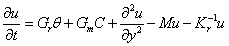(9)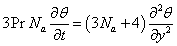(10)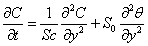(11)

The transformed initial and boundary conditions are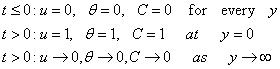(12)

#### Method of Solution

The equations (9) to (11) are nonlinear, coupled partial differential equations, so we want to solve them by using Laplace transform technique. Taking Laplace transform, the equations (9), (10) and (11) reduce to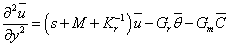(13)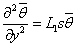(14)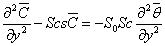(15)

Where‘s’ is the Laplace transform parameter. The boundary condition (12) reduces to the following form after applying Laplace transform.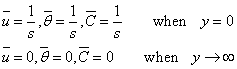(16)

Solving (13), (14) and (15) with boundary condition (16) we get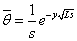(17)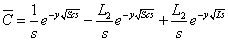(18)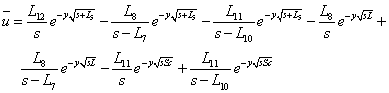(19)

Inverting the equations (17), (18) and (19) we get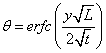(20)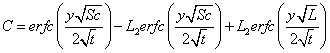(21)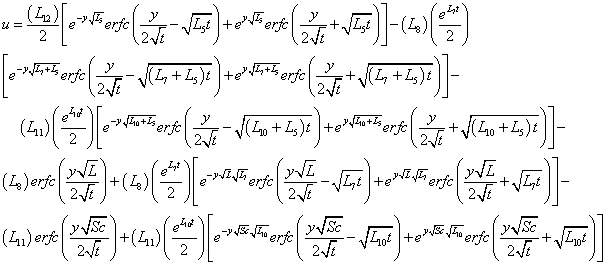(22)

The Skin friction at the surface of the plate is given by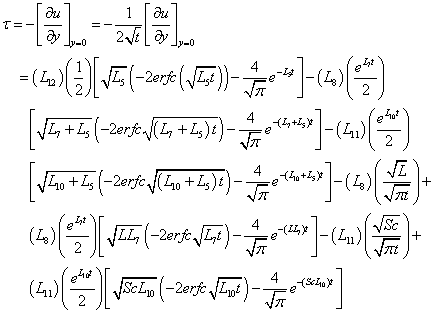(23)

The Nusselt number and Sherwood number at the plate are respectively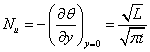(24)

and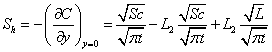(24)

#### Result and Discussion

To discuss the physical implication of various parameters involved in the results (20) - (25), the numerical calculation has been carried out for the distributions of velocity, temperature, concentration, Skin friction, Nusselt number and Sherwood number. The effects of various physical parameters on these flow quantities such as Hartmann number M, Prandtl number Pr, Soret number S0, Schmidt number Sc, Permeability parameter Kr, Grashof number Gr, modified Grashof number Gm and Radiation Parameter Na are studied though graphs. The concentration profiles are plotted in Figure 1 for various values of Schmidt number Sc. From this figure, it is noticed that the concentration decreases with an increase in the values of the Schmidt number Sc. A comparison of curves in the figure shows a decrease in concentration with an increase in Schmidt number Sc. Actually it is true, since the increase of Sc means decrease of molecular diffusivity and therefore decreases in concentration boundary layer. The effects of increasing the Soret number S0 on the species concentration profiles have been shown in Figure 2. From this figure, it is noticed that an increase in Soret number S0 results an increase in the concentration profiles. Figure 3 revels the temperature profiles for different values of Prandtl number Pr. It is observed that the temperature decrease as an increase in the values of Prandtl number Pr. The reason is that smaller values of Prandtl number are equivalent to increase in the thermal conductivity of the fluid and therefore heat is able to diffuse away from the heated surface extra rapidly for higher values of Pr (Appendix). Hence, in the case of larger Prandtl number the thermal boundary layer is thinner and the rate of heat transfer is reduced. Figure 4 shows the temperature profile for different values of Radiation Parameter Na. From this figure it is noticed that an increase in the values of Na results a decrease in the temperature profiles. The effect of Grashof number Gr on velocity is presented in Figure 5. It is observed that an increase in Gr leads to a rise in the velocity boundary layer. Figure 6 shows the velocity profile for different values of modified Grashof number. From this figure it is observed that an increase in the values of modified Grashof number Gm results in increase in the velocity profiles. Figure 7 shows the velocity profiles for different values of radiation parameter Na. From this figure it is notice that velocity decreases with increase in Na. Figure 8 revels the effect of Prandtl number Pr on the velocity profile. It is evident from the figure that the velocity decreases with an increase in Pr. Figure 9 illustrates the velocity profiles for different values of Schmidt number Sc. It has been observed that the velocity decreases with increase in Sc. Figure 10 shows the velocity profiles for different values of Soret number S0. It was found that an increase in the value of S0 leads to an increase in the velocity distribution across the boundary layer. This is true, as the S0 increases, small light molecules and large heavy molecules get separated under a temperature gradient, which intern increases the velocity of a fluid. Figure 11 illustrates the velocity profiles for different values of Hartmann number M. From this figure it is notice that velocity decrease with an increase in Hartmann number M. Figure 12 reveals the effect of time t on the transient velocity profiles. It is evident from the figure that the velocity decreases with increase in t. The velocity profiles are plotted in Figure 13 for various values of permeability parameter Kr From this figure, it is noticed that the velocity increases with the increase in the values of the permeability parameter Kr. Physically, an increase in the permeability of porous medium leads to rise, in the flow of fluid during it. When the holes of the porous medium become large, the resistance of the medium may be neglected. A similar approach is noticed with Raju et al. . Figure 14 shows Sherwood number is presented against time t for different values of Schmidt numbers. We observed that Sherwood number increases with increasing Schmidt numbers Sc. Figure 15 shows the Sherwood number (Sh) on the porous plate for different values of Soret number S0. The result display that an increase in the value of S0 results an decrease in the Sherwood number. Figure 16 presents the variation of the Nusselt number Nu against time t for various values of Prandtl number Pr. From this figure we notice that Nusselt number increases when the values of Prandtl number Pr increase. Figure 17 illustrates the Nusselt number for different values of radiation parameter Na. From this figure it is noticed that Nusselt number increases with increase in Na. Figures 18 and 19 depicts skin-friction against time t for different values of Grashof number Gr and modified Grashof number Gm. From these figures it is notice that Skin-friction increases with an increase Gr and Gm. Figure 20 depict skin-friction against time t for different values of Hartmann number M. It is observed that the skin friction decreases with increase in Hartmann number M. Figures 21-23 depicts skinfriction against time t for different values of Permeability parameter Kr, Schmidt number Sc and Prandtl number Pr. From these figures it notice that skin-friction increases with an increases Kr , Sc and Pr.

#### Conclusion

In this paper a theoretical examination has been carried out to study thermal diffusion on MHD free convective radiating flow more an impulsively started vertical plate embedded in a porous medium. Solutions for the model has been derived by using Laplace transform technique. Some conclusions of the study are as follow:

• Concentration distributed is observed to decrease with increase in Schmidt number and it increases with increase in Soret number.

• Temperature decreases with increase in Pr and Na.

• Velocity increases with increase in Gr, Gm, S0 and Kr while it decreases with increase in Na, Pr, Sc, M and t.

• Sherwood number increase with increase in Sc and decrease with increase S0.

• Nusselt number increases with increase in Pr and Na.

• Skin-friction increases with an increase in Gr, Gm, Kr, Sc and Pr and decreases with increase in M.

#### References

Select your language of interest to view the total content in your interested language

### Article Usage

• Total views: 8912
• [From(publication date):
March-2016 - Nov 15, 2019]
• Breakdown by view type
• HTML page views : 8715Can't read the image? click here to refresh
###### Peer Reviewed Journals

Make the best use of Scientific Research and information from our 700 + peer reviewed, Open Access Journals

### Clinical & Medical Journals

International Conferences 2019-20

Meet Inspiring Speakers and Experts at our 3000+ Global Annual Meetings

Top